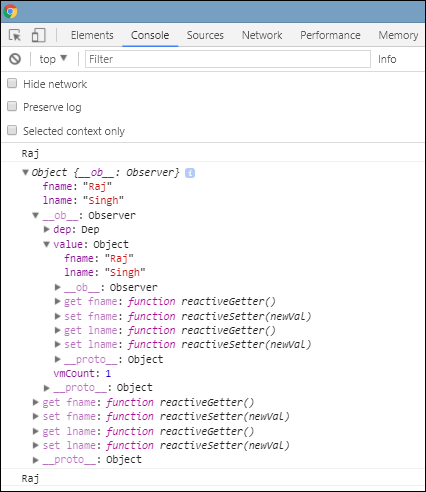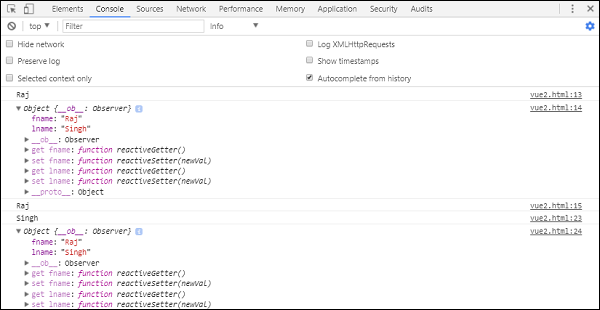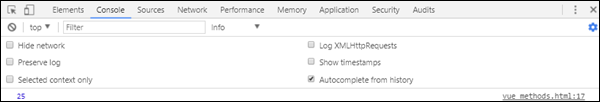# VueJS - Instances

To start with VueJS, we need to create the instance of Vue, which is called the root Vue Instance.

## Syntax

```var app = new Vue({
// options
})
```

Let us look at an example to understand what needs to be part of the Vue constructor.

```<html>
<title>VueJs Instance</title>
<script type = "text/javascript" src = "js/vue.js"></script>
<body>
<div id = "vue_det">
<h1>Firstname : {{firstname}}</h1>
<h1>Lastname : {{lastname}}</h1>
<h1>{{mydetails()}}</h1>
</div>
<script type = "text/javascript" src = "js/vue_instance.js"></script>
</body>
</html>
```

### vue_instance.js

```var  vm = new Vue({
el: '#vue_det',
data: {
firstname : "Ria",
lastname  : "Singh",
},
methods: {
mydetails : function() {
return "I am "+this.firstname +" "+ this.lastname;
}
}
})
```

For Vue, there is a parameter called el. It takes the id of the DOM element. In the above example, we have the id #vue_det. It is the id of the div element, which is present in .html.

```<div id = "vue_det"></div>
```

Now, whatever we are going to do will affect the div element and nothing outside it.

Next, we have defined the data object. It has value firstname, lastname, and address.

The same is assigned inside the div. For example,

```<div id = "vue_det">
<h1>Firstname : {{firstname}}</h1>
<h1>Lastname : {{lastname}}</h1>
</div>
```

The Firstname : {{firstname}} value will be replaced inside the interpolation, i.e. {{}} with the value assigned in the data object, i.e. Ria. The same goes for last name.

Next, we have methods where we have defined a function mydetails and a returning value. It is assigned inside the div as

```<h1>{{mydetails()}}</h1>
```

Hence, inside {{} } the function mydetails is called. The value returned in the Vue instance will be printed inside {{}}. Check the output for reference.

## OutputNow, we need to pass options to the Vue constructor which is mainly data, template, element to mount on, methods, callbacks, etc.

Let us take a look at the options to be passed to the Vue.

#data − This type of data can be an object or a function. Vue converts its properties to getters/setters to make it reactive.

Let’s take a look at how the data is passed in the options.

## Example

```<html>
<title>VueJs Introduction</title>
<script type = "text/javascript" src = "js/vue.js"></script>
<body>
<script type = "text/javascript">
var _obj = { fname: "Raj", lname: "Singh"}

// direct instance creation
var vm = new Vue({
data: _obj
});
console.log(vm.fname);
console.log(vm.\$data);
console.log(vm.\$data.fname);
</script>
</body>
</html>
```

## Outputconsole.log(vm.fname); // prints Raj

console.log(vm.\$data); prints the full object as shown above

console.log(vm.\$data.fname); // prints Raj

If there is a component, the data object has to be referred from a function as shown in the following code.

```<html>
<title>VueJs Introduction</title>
<script type = "text/javascript" src = "js/vue.js"></script>
<body>
<script type = "text/javascript">
var _obj = { fname: "Raj", lname: "Singh"};

// direct instance creation
var vm = new Vue({
data: _obj
});
console.log(vm.fname);
console.log(vm.\$data);
console.log(vm.\$data.fname);

// must use function when in Vue.extend()
var Component = Vue.extend({
data: function () {
return _obj
}
});
var myComponentInstance = new Component();
console.log(myComponentInstance.lname);
console.log(myComponentInstance.\$data);
</script>
</body>
</html>
```

In case of a component, the data is a function, which is used with Vue.extend as shown above. The data is a function. For example,

```data: function () {
return _obj
}
```

To refer to the data from the component, we need to create an instance of it. For example,

```var myComponentInstance = new Component();
```

To fetch the details from the data, we need to do the same as we did with the parent component above. For example.

```console.log(myComponentInstance.lname);
console.log(myComponentInstance.\$data);
```

Following are the details displayed in the browser.Props − Type for props is an array of string or object. It takes an array-based or object-based syntax. They are said to be attributes used to accept data from the parent component.

## Example 1

```Vue.component('props-demo-simple', {
props: ['size', 'myMessage']
})
```

## Example 2

```Vue.component('props-demo-advanced', {
props: {
// just type check
height: Number,

// type check plus other validations
age: {
type: Number,
default: 0,
required: true,
validator: function (value) {
return value >= 0
}
}
}
})
```

propsData − This is used for unit testing.

Type − array of string. For example, { [key: string]: any }. It needs to be passed during the creation of Vue instance.

## Example

```var Comp = Vue.extend({
props: ['msg'],
template: '<div>{{ msg }}</div>'
})
var vm = new Comp({
propsData: {
msg: 'hello'
}
})
```

Computed − Type: { [key: string]: Function | { get: Function, set: Function } }

## Example

```<html>
<title>VueJs Introduction</title>
<script type = "text/javascript" src = "js/vue.js"></script>
<body>
<script type = "text/javascript">
var vm = new Vue({
data: { a: 2 },
computed: {

// get only, just need a function
aSum: function () {
return this.a + 2;
},

// both get and set
aSquare: {
get: function () {
return this.a*this.a;
},
set: function (v) {
this.a = v*2;
}
}
}
})
console.log(vm.aSquare);  // -> 4
vm.aSquare = 3;
console.log(vm.a);       // -> 6
console.log(vm.aSum); // -> 8
</script>
</body>
</html>
```

Computed has two functions aSum and aSquare.

Function aSum just returns this.a+2. Function aSquare again two functions get and set.

Variable vm is an instance of Vue and it calls aSquare and aSum. Also vm.aSquare = 3 calls the set function from aSquare and vm.aSquare calls the get function. We can check the output in the browser which looks like the following screenshot.Methods − Methods are to be included with the Vue instance as shown in the following code. We can access the function using the Vue object.

```<html>
<title>VueJs Introduction</title>
<script type = "text/javascript" src = "js/vue.js"></script>
<body>
<script type = "text/javascript">
var vm = new Vue({
data: { a: 5 },
methods: {
asquare: function () {
this.a *= this.a;
}
}
})
vm.asquare();
console.log(vm.a); // 25
</script>
</body>
</html>
```

Methods are part of the Vue constructor. Let us make a call to the method using the Vue object vm.asquare (), the value of the property a is updated in the asquare function. The value of a is changed from 1 to 25, and the same is seen reflected in the following browser console.